# Consider the initial value problem: y′′+16y=e^-t, y(0)= y_0, y′(0)=y'_0 Suppose we know that y(t)→0y as t→∞. Determine the solution and the initial conditions.Find:y(t)y(0)y'(0)

Question
191 views

Consider the initial value problem: y′′+16y=e^-t, y(0)= y_0, y′(0)=y'_0

Suppose we know that y(t)→0y as t→∞. Determine the solution and the initial conditions.

Find:

y(t)

y(0)

y'(0)

check_circle

Step 1

We are given the differential equation by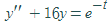.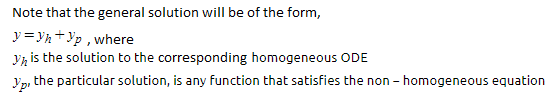So, first, we will solve for yh and then yp.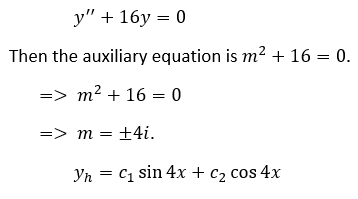Step 2

Now, the particular solution.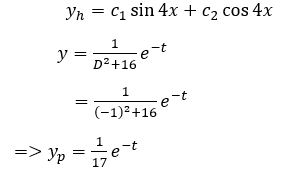So, the solution of the given differential equation is given by,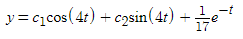.

...

### Want to see the full answer?

See Solution

#### Want to see this answer and more?

Solutions are written by subject experts who are available 24/7. Questions are typically answered within 1 hour.*

See Solution
*Response times may vary by subject and question.
Tagged in